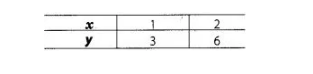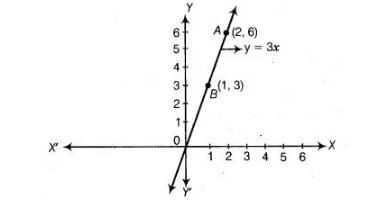# Write the linear equation such that`
Question:

Write the linear equation such that each point on its graph has an ordinate 3 times its abscissa.

Solution:

Let the abscissa of the point be x,
According to the question, Ordinate (y) = 3 x Abscissa => y=3x When x = 1, then y = 3 x 1 = 3 and when x = 2, then y = 3 x 2 = 6.Here, we find two points $A(1,3)$ and $B(2,6)$. So, draw the graph by plotting the points and joining the line $A B$.Hence, y = 3x is the required equation such that each point on its graph has an ordinate 3 times its abscissa.